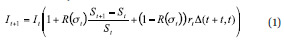Optimal Design Of Risk Control Strategy Indexes

August 17, 2012

Index Methodology
We intend to derive a sophisticated analytical understanding of the risk/return profile of risk-control strategy indexes and to verify the key results by numerical backtests. In essence, we assume a strategy index that invests in the holdings of a standard equity blue-chip index with value St at time t and a money market instrument, where in principle both investments can be long or short.

The appendixes of this paper are available online.1 There, we derive a complex mathematical description of this type of strategy index that is based on the well-known Brownian-motion model for the underlying equity index, which is commonly used in option pricing. In essence, this model is based on the assumption of equity returns following a normal distribution. Since real-world equity returns typically show heavy tails—which means that real-world equity markets are riskier than a normal distribution would predict—we will use numerical simulations based on real-world equity returns to verify the theoretical results based on this model.

As a general index concept, we consider a strategy index It that uses equity volatility ?t observed in the market at time t as an asset allocation signal in the sense that it invests a portion of R(?t) into the underlying equity index St and a portion 1-R(?t) into the money market at rate rt . We will refer to the equity portfolio weight R(?t) as the response function, since it determines the amount of equity held in the index portfolio depending on the current level of equity volatility.

The index-update formula that index providers use for this type of strategy index basically states that the index return is the weighted average of the return on the equity investment and the money market investment. It takes the form:where ?(t+1, t) denotes the time between date t and t+1 in the respective date-count convention. In principle, leveraged equity indexes and target volatility indexes are calculated according to formula (1), although they are quite different in terms of the way the size of the equity investment R(?t) is determined (as we will elaborate later) and also in terms of the rebalancing frequency: Leveraged indexes are typically rebalanced periodically (i.e., daily, weekly or monthly), whereas most target volatility indexes use a triggered rebalancing (i.e., rebalancing takes place when the actual weight of the equity investment in the index deviates by more than a predefined threshold from the desired weight R(?t)). Nevertheless, both index concepts can be analyzed within the same mathematical framework and can be improved by implementing an optimal risk-control mechanism in a similar way.